# Finding inverse of a matrix using Gauss – Jordan Method | Set 2

Given a Matrix, the task is to find the inverse of this Matrix using the Gauss-Jordan method.

What is matrix?

Matrix is an ordered rectangular array of numbers.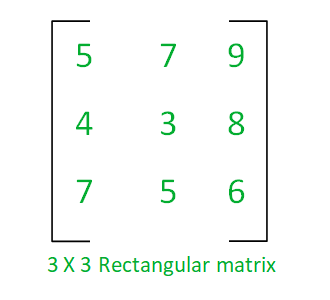Operations that can be performed on a matrix are: Addition, Subtraction, Multiplication or Transpose of matrix etc.

### Inverse of a matrix:

Given a square matrix A, which is non-singular (means the Determinant of A is nonzero); Then there exists a matrixwhich is called inverse of matrix A.

The inverse of a matrix is only possible when such properties hold:

1. The matrix must be a square matrix.
2. The matrix must be a non-singular matrix and,
3. There exist an Identity matrix I for whichIn general, the inverse of n X n matrix A can be found using this simple formula: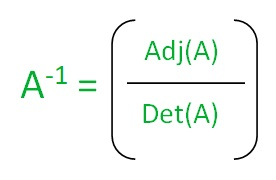where, Adj(A) denotes the adjoint of a matrix and, Det(A) is Determinant of matrix A.

### Methods for finding Inverse of Matrix:

Finding the inverse of a 2×2 matrix is a simple task, but for finding the inverse of larger matrix (like 3×3, 4×4, etc) is a tough task, So the following methods can be used:

1. Elementary Row Operation (Gauss-Jordan Method) (Efficient)
2. Minors, Cofactors and Ad-jugate Method (Inefficient)

### Elementary Row Operation (Gauss – Jordan Method):

Gauss-Jordan Method is a variant of Gaussian elimination in which row reduction operation is performed to find the inverse of a matrix.

Steps to find the inverse of a matrix using Gauss-Jordan method:

In order to find the inverse of the matrix following steps need to be followed:

1. Form the augmented matrix by the identity matrix.
2. Perform the row reduction operation on this augmented matrix to generate a row reduced echelon form of the matrix.
3. The following row operations are performed on augmented matrix when required:
• Interchange any two row.
• Multiply each element of row by a non-zero integer.
• Replace a row by the sum of itself and a constant multiple of another row of the matrix.

Example:

•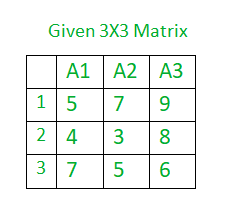• Augmented Matrix is formed as A:B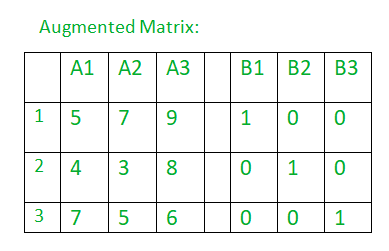• After applying the Gauss-Jordan elimination method: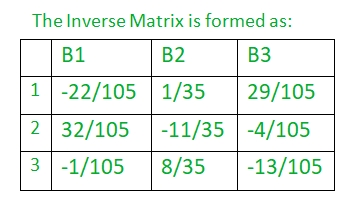Below is the C++ program to find the inverse of a matrix using the Gauss-Jordan method:

 `// C++ program to find the inverse of Matrix. ` ` `  `#include ` `#include ` `using` `namespace` `std; ` ` `  `// Function to Print matrix. ` `void` `PrintMatrix(``float` `ar[], ``int` `n, ``int` `m) ` `{ ` `    ``for` `(``int` `i = 0; i < n; i++) { ` `        ``for` `(``int` `j = 0; j < m; j++) { ` `            ``cout << ar[i][j] << ``"  "``; ` `        ``} ` `        ``printf``(``"\n"``); ` `    ``} ` `    ``return``; ` `} ` ` `  `// Function to Print inverse matrix ` `void` `PrintInverse(``float` `ar[], ``int` `n, ``int` `m) ` `{ ` `    ``for` `(``int` `i = 0; i < n; i++) { ` `        ``for` `(``int` `j = n; j < m; j++) { ` `            ``printf``(``"%.3f  "``, ar[i][j]); ` `        ``} ` `        ``printf``(``"\n"``); ` `    ``} ` `    ``return``; ` `} ` ` `  `// Function to perform the inverse operation on the matrix. ` `void` `InverseOfMatrix(``float` `matrix[], ``int` `order) ` `{ ` `    ``// Matrix Declaration. ` ` `  `    ``float` `temp; ` ` `  `    ``// PrintMatrix function to print the element ` `    ``// of the matrix. ` `    ``printf``(``"=== Matrix ===\n"``); ` `    ``PrintMatrix(matrix, order, order); ` ` `  `    ``// Create the augmented matrix ` `    ``// Add the identity matrix ` `    ``// of order at the end of original matrix. ` `    ``for` `(``int` `i = 0; i < order; i++) { ` ` `  `        ``for` `(``int` `j = 0; j < 2 * order; j++) { ` ` `  `            ``// Add '1' at the diagonal places of ` `            ``// the matrix to create a identity matirx ` `            ``if` `(j == (i + order)) ` `                ``matrix[i][j] = 1; ` `        ``} ` `    ``} ` ` `  `    ``// Interchange the row of matrix, ` `    ``// interchanging of row will start from the last row ` `    ``for` `(``int` `i = order - 1; i > 0; i--) { ` ` `  `        ``// Swapping each and every element of the two rows ` `        ``// if (matrix[i - 1] < matrix[i]) ` `        ``// for (int j = 0; j < 2 * order; j++) { ` `        ``// ` `        ``//        // Swapping of the row, if above ` `        ``//        // condition satisfied. ` `        ``// temp = matrix[i][j]; ` `        ``// matrix[i][j] = matrix[i - 1][j]; ` `        ``// matrix[i - 1][j] = temp; ` `        ``//    } ` ` `  `        ``// Directly swapping the rows using pointers saves time ` ` `  `        ``if` `(matrix[i - 1] < matrix[i]) { ` `            ``float``* temp = matrix[i]; ` `            ``matrix[i] = matrix[i - 1]; ` `            ``matrix[i - 1] = temp; ` `        ``} ` `    ``} ` ` `  `    ``// Print matrix after interchange operations. ` `    ``printf``(``"\n=== Augmented Matrix ===\n"``); ` `    ``PrintMatrix(matrix, order, order * 2); ` ` `  `    ``// Replace a row by sum of itself and a ` `    ``// constant multiple of another row of the matrix ` `    ``for` `(``int` `i = 0; i < order; i++) { ` ` `  `        ``for` `(``int` `j = 0; j < order; j++) { ` ` `  `            ``if` `(j != i) { ` ` `  `                ``temp = matrix[j][i] / matrix[i][i]; ` `                ``for` `(``int` `k = 0; k < 2 * order; k++) { ` ` `  `                    ``matrix[j][k] -= matrix[i][k] * temp; ` `                ``} ` `            ``} ` `        ``} ` `    ``} ` ` `  `    ``// Multiply each row by a nonzero integer. ` `    ``// Divide row element by the diagonal element ` `    ``for` `(``int` `i = 0; i < order; i++) { ` ` `  `        ``temp = matrix[i][i]; ` `        ``for` `(``int` `j = 0; j < 2 * order; j++) { ` ` `  `            ``matrix[i][j] = matrix[i][j] / temp; ` `        ``} ` `    ``} ` ` `  `    ``// print the resultant Inverse matrix. ` `    ``printf``(``"\n=== Inverse Matrix ===\n"``); ` `    ``PrintInverse(matrix, order, 2 * order); ` ` `  `    ``return``; ` `} ` ` `  `// Driver code ` `int` `main() ` `{ ` `    ``int` `order; ` ` `  `    ``// Order of the matrix ` `    ``// The matrix must be a square a matrix ` `    ``order = 3; ` `    ``float` `matrix = { { 5, 7, 9 }, ` `                             ``{ 4, 3, 8 }, ` `                             ``{ 7, 5, 6 }, ` `                             ``{ 0 } }; ` ` `  `    ``// Get the inverse of matrix ` `    ``InverseOfMatrix(matrix, order); ` ` `  `    ``return` `0; ` `} `

Output:

```=== Matrix ===
5  7  9
4  3  8
7  5  6

=== Augmented Matrix ===
7  5  6  0  0  1
5  7  9  1  0  0
4  3  8  0  1  0

=== Inverse Matrix ===
-0.210  0.029  0.276
0.305  -0.314  -0.038
-0.010  0.229  -0.124
```

Attention reader! Don’t stop learning now. Get hold of all the important DSA concepts with the DSA Self Paced Course at a student-friendly price and become industry ready.

My Personal Notes arrow_drop_upCheck out this Author's contributed articles.

If you like GeeksforGeeks and would like to contribute, you can also write an article using contribute.geeksforgeeks.org or mail your article to contribute@geeksforgeeks.org. See your article appearing on the GeeksforGeeks main page and help other Geeks.

Please Improve this article if you find anything incorrect by clicking on the "Improve Article" button below.